# Chapter 4 Partial Differential Equations

## 4.1  Examples of Partial Differential Equations

Partial differential equations arise in almost every problem that exists in multi-dimensional space. The gradient operator of vector calculus is (in three dimensional space)
 $\nabla =\left(\frac{\partial }{\partial x},\frac{\partial }{\partial y},\frac{\partial }{\partial z}\right),$ $\left(4.1\right)$
a partial differential operator.
Partial differential equations also arise when there is time-dependent behavior within a domain that is one-dimensional in space. Then the two dimensions (independent variables) are $x$ and $t$.
Probably the most important part of computational science and engineering is formulating the calculation in terms of partial differential equations. Once a situation is properly formulated, the numerical techniques we are studying can be applied. But turning a problem in the real world into partial differential equations representing it, requires deep knowledge. This section can give only a few examples.

### 4.1.1  Fluid Flow

The flow of a substance that can be considered to be a continuum fluid, such as water, or (collisional) gas, is governed by a hierarchy of equations. They are fundamentally conservation equations of substance (e.g. mass), of momentum, and of energy. The momentum conservation equation is often called the Navier-Stokes equation. It relies on the other equations. Let's derive the conservation of substance or continuity equation.
Consider a substance with density $\mathit{\rho }\left(\mathbit{x}\right)$ which we can consider to be the substance's mass per unit volume (but could alternatively be number of particles per unit volume or electric charge per unit volume). Suppose that this substance has a volumetric source density $S\left(\mathbit{x}\right)$. This quantity denotes the amount (mass) of substance that is created per unit time per unit volume at position $\mathbit{x}$. Such creation might be by chemical reaction (e.g. producing the substance CO${}_{2}$ from reaction of CO and O${}_{2}$) or it might be by nuclear reaction (e.g. producing Xe${}^{135}$ by fission of uranium). The source could also be negative, corresponding to destruction of the substance, for example radioactive disintegration of Xe${}^{135}$, or a balance between creation and destruction. Apart from the processes represented by $S$, however, the substance is conserved (neither created nor destroyed). If the substance is able to flow, and has a velocity $\mathbit{v}\left(\mathbit{x}\right)$, then it can move around and as it does so it gives rise to a flux density of substance $\mathit{\rho }\mathbit{v}$ (mass per unit area per unit time). [Flux density represents the amount (mass) of substance per unit time carried by the flow across unit area with normal in a certain direction; it is therefore a vector quantity.] Across any small surface (element) $\mathbit{dA}$ the flux (mass per unit time) is equal to $\mathit{\rho }\mathbit{v}.\mathbit{dA}$, as illustrated in Fig. 4.1.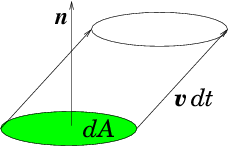Figure 4.1: The elementary volume of fluid crossing a surface element $\mathit{dA}$ in time element $\mathit{dt}$ is $\mathit{dt}\mathbit{v}.\stackrel{^}{\mathbit{n}}\mathit{dA}=\mathit{dt}\mathbit{v}.\mathbit{dA}$. So the mass per unit time is $\mathit{\rho }\mathbit{v}.\mathbit{dA}$.
Mass conservation is then described by considering some control volume $V$ whose surface is $\partial V$. For any such volume, the rate of increase of the total amount of substance within the volume must be equal to the total source density within the volume plus the amount flowing in across its surface:
 $\frac{\partial }{\partial t}{\int }_{V}\mathit{\rho }{d}^{3}x={\int }_{V}S{d}^{3}x-{\int }_{\partial V}\mathit{\rho }\mathbit{v}.\mathbit{dA}.$ $\left(4.2\right)$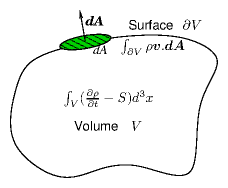Figure 4.2: Conservation integrals over arbitrary volume $V$ with surface $\partial V$.
Using Gauss's (divergence) theorem, illustrated in Fig. 4.2, that for any vector field $\mathbit{u}$ (here $\mathbit{u}=\mathit{\rho }\mathbit{v}$), the integral over a closed volume of the divergence is equal to the surface integral of the vector:
 ${\int }_{V}\nabla .\mathbit{u}\mathrm{ }{d}^{3}x={\int }_{\partial V}\mathbit{u}.\mathbit{dA},$ $\left(4.3\right)$
the surface term can be converted to a volume integral and the mass conservation equation becomes:
 ${\int }_{V}\left(\frac{\partial \mathit{\rho }}{\partial t}+\nabla .\left(\mathit{\rho }\mathbit{v}\right)-S\right){d}^{3}x=0.$ $\left(4.4\right)$
This identity applies for any volume $V$. The only way that can be true is if the integrand is everywhere equal to zero:
 $\frac{\partial \mathit{\rho }}{\partial t}+\nabla .\left(\mathit{\rho }\mathbit{v}\right)-S=0.$ $\left(4.5\right)$
This is usually called the "continuity" equation. Sometimes, arguably more physically, it is called the "advection" equation. It is a partial differential equation in three space and one time variables.
We'll discuss the rest of the fluid equations later; but for now consider a steady state $\frac{\partial }{\partial t}=0$, in which the velocity $\mathbit{v}\left(\mathbit{x}\right)$ is prescribed everywhere, illustrated in Fig. 4.3. The resulting partial differential equation is
 $\nabla .\left(\mathit{\rho }\mathbit{v}\right)=\mathbit{v}.\nabla \mathit{\rho }+\mathit{\rho }\nabla .\mathbit{v}={v}_{x}\frac{\partial \mathit{\rho }}{\partial x}+{v}_{y}\frac{\partial \mathit{\rho }}{\partial y}+{v}_{z}\frac{\partial \mathit{\rho }}{\partial z}+G\left(\mathbit{x}\right)\mathit{\rho }=S,$ $\left(4.6\right)$
where the components of the velocity ${v}_{x}$, ${v}_{y}$, ${v}_{z}$ and the divergence of the velocity, $G=\nabla .\mathbit{v}$, are prescribed functions of position.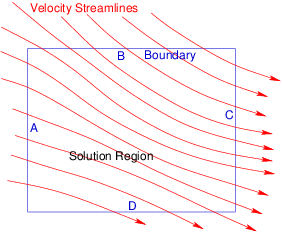Figure 4.3: The advection equation with prescribed velocity $\mathbit{v}$ amounts to integration along the streamlines. Conditions ("initial" conditions) can be applied along the solution region boundaries A and B, but not then along C and D.
From the point of view of solving for $\mathit{\rho }$ as a function of space, this is a linear first-order partial differential equation in three dimensions, with variable (i.e. non-uniform) coefficients ${v}_{x}$, ${v}_{y}$, ${v}_{z}$, and $G$. It is inhomogeneous if $S\ne 0$, but homogeneous if $S=0$ everywhere.

### 4.1.2  Diffusion

When a substance such as a gas diffuses through a some other medium, for example a porous solid, or another (flow free) gas, the flux density $\mathit{\rho }\mathbit{v}$ of the substance is frequently proportional to the gradient of its density $\mathit{\rho }$:
 $\mathit{\rho }\mathbit{v}=-D\nabla \mathit{\rho },$ $\left(4.7\right)$
where $D$ is the diffusion coefficient.
For such a diffusion problem, we can eliminate the velocity from the continuity equation (4.5) and obtain the diffusion equation:
 $\frac{\partial \mathit{\rho }}{\partial t}-\nabla .\left(D\nabla \mathit{\rho }\right)-S=0.$ $\left(4.8\right)$
This is a linear partial differential equation that is second order in the spatial derivatives (e.g. if $D$ is uniform the second term becomes Laplacian $-D{\nabla }^{2}\mathit{\rho }$); but it is first order in time.

### 4.1.3  Waves

The equation that governs waves in one dimension, for example small-amplitude compressional vibrations of a column of air in a pipe, or transverse vibrations of a stretched string or a rigid rod, is
 $\frac{{\partial }^{2}\mathit{\psi }}{\partial {t}^{2}}={c}_{s}^{2}\frac{{\partial }^{2}\mathit{\psi }}{\partial {x}^{2}},$ $\left(4.9\right)$
where ${c}_{s}$ is the wave (sound) speed, and $\mathit{\psi }$ represents the wave displacement or perturbed quantity (e.g. pressure). The wave equation is second order in space and time, and linear (provided ${c}_{s}$ does not depend on $\mathit{\psi }$).

### 4.1.4  Electromagnetism

Maxwell's equations of electromagnetism, relating the electric and magnetic fields $\mathbit{E}$, and $\mathbit{B}$, to the charge density ${\mathit{\rho }}_{q}$, and the electric current density $\mathbit{j}$, in the absence of dielectric or magnetic materials are:
 $\begin{array}{cccc}\multicolumn{1}{c}{}& \hfill & \nabla .\mathbit{E}={\mathit{\rho }}_{q}/{\mathit{\epsilon }}_{0}\hfill & \hfill \left(4.10\right)\\ \multicolumn{1}{c}{}& \hfill & \nabla ×\mathbit{E}=-\frac{\partial \mathbit{B}}{\partial t}\hfill \\ \multicolumn{1}{c}{}& \hfill & \nabla .\mathbit{B}=0\hfill \\ \multicolumn{1}{c}{}& \hfill & \nabla ×\mathbit{B}={\mathit{\mu }}_{0}\mathbit{j}+{\mathit{\mu }}_{0}{\mathit{\epsilon }}_{0}\frac{\partial \mathbit{E}}{\partial t}\hfill \end{array}$

where ${\mathit{\epsilon }}_{0}=8.85×{10}^{-12}$ F/m is the permittivity of free space, ${\mathit{\mu }}_{0}=4\mathit{\pi }×{10}^{-7}$ H/m is the permeability of free space. These fundamental constants satisfy ${\mathit{\mu }}_{0}{\mathit{\epsilon }}_{0}=1/{c}^{2}$, where $c$ is the speed of light.
These are a system of partial differential equations. There appear to be eight in all because the curl equations are vector equations (three equations in one) and the divergence equations are single equations. However, there's some built-in redundancy in the equations that reduces there effective number to six (equal to the number of dependent variables in the components of the electric and magnetic fields).
Only rarely does one solve the full set of equations numerically. More usually one is interested in simplified special cases. For example, if time dependence can be ignored, then $\nabla ×\mathbit{E}=0$, which is a sufficient condition to allow the electric field to be expressed as the gradient of a scalar potential $\mathbit{E}=-\nabla \mathit{\phi }$. In that case, the potential satisfies
 $-\nabla .\mathbit{E}=-\nabla .\left(-\nabla \mathit{\phi }\right)={\nabla }^{2}\mathit{\phi }=\frac{{\partial }^{2}\mathit{\phi }}{\partial {x}^{2}}+\frac{{\partial }^{2}\mathit{\phi }}{\partial {y}^{2}}+\frac{{\partial }^{2}\mathit{\phi }}{\partial {z}^{2}}=-{\mathit{\rho }}_{q}/{\mathit{\epsilon }}_{0},$ $\left(4.11\right)$
which is Poisson's equation${}^{23}$. Poisson's equation sets a second order differential, the Laplacian (${\nabla }^{2}$) of the potential equal to a (presumably prescribed) function $-{\mathit{\rho }}_{q}/{\mathit{\epsilon }}_{0}$.

## 4.2  Classification of Partial Differential Equations

In the theory of partial differential equations, there are three types of differential equation. They are called: Hyperbolic, Parabolic, and Elliptic. To understand this classification rigorously would take us far beyond our scope, but it is important for computation because the methods of solving the different types of equation are different.
For our purposes, the classification can be considered as follows for second order equations. Write the general linear second-order partial differential equation governing dependent variable $\mathit{\psi }$ for independent variables ${x}_{i}$ as
 $\sum _{i,j}{c}_{\mathit{ij}}\frac{\partial }{\partial {x}_{i}}\frac{\partial }{\partial {x}_{j}}\mathit{\psi }+\sum _{i}{c}_{i}\frac{\partial }{\partial {x}_{i}}\mathit{\psi }+c\mathit{\psi }=\mathit{const}.$ $\left(4.12\right)$
A specific example might be
 $A\frac{{\partial }^{2}}{\partial {x}^{2}}\mathit{\psi }+2B\frac{{\partial }^{2}}{\partial x\partial y}\mathit{\psi }+C\frac{{\partial }^{2}}{\partial {y}^{2}}\mathit{\psi }=0.$ $\left(4.13\right)$
Consider the coefficients ${c}_{\mathit{ij}}$, and regard them as defining a surface in the multidimensional space via the quadratic form associated with them:
 $\sum _{i,j}{c}_{\mathit{ij}}{x}_{i}{x}_{j}+\sum _{i}{c}_{i}{x}_{i}=\mathit{const}.$ $\left(4.14\right)$
Specifically:
 ${\mathit{Ax}}^{2}+2B\mathit{xy}+{\mathit{Cy}}^{2}=\mathit{const}.$ $\left(4.15\right)$
Then the partial differential equation is hyperbolic, parabolic, or elliptic according to whether the surface so defined is itself hyperbolic, parabolic, or elliptic.
For our two-dimensional specific example, the surface is
Hyperbolic
if ${B}^{2}-\mathit{AC}>0$; for example $B=0$, $C=-A$, ${x}^{2}-{y}^{2}=\mathit{const}.$
Parabolic
if ${B}^{2}-\mathit{AC}=0$; for example $B=C=0$, ${x}^{2}-y=\mathit{const}.$
Elliptic
if ${B}^{2}-\mathit{AC}<0$; for example $B=0$, $C=A$, ${x}^{2}+{y}^{2}=\mathit{const}.$
And so is the equation. The examples we have given previously illustrate characteristically these different types.
The wave equation is hyperbolic.
The diffusion equation is parabolic.
The Poisson equation is elliptic.
The first order equation for fluid flow with prescribed velocity (advection equation) is ambiguous under this classification because the surface for a first order form is a plane. But a plane can be considered a degenerate hyperbola, because it extends to infinity (unlike an ellipse which is finite in extent). The first order system for one scalar dependent variable is always hyperbolic.${}^{24}$
Loosely speaking, second order hyperbolic equations are wave-like, and elliptic equations are steady-flux-conservation-like. A hyperbolic or parabolic equation generally has at least one independent variable that is like time, as well as others that are like space. An elliptic problem is more like a steady (non-time-varying) problem in multiple space dimensions. The classification is more fundamentally to do with whether a problem of order $N$ with $N$ boundary conditions on a single surface (the multidimensional equivalent of an initial value problem) can be solved or not. Such a problem is called a Cauchy problem. Generally, hyperbolic equations are those for which the Cauchy problem can be solved; for elliptic equations it cannot.
The most important distinction from the viewpoint of numerical solution of partial differential equations is with respect to the boundary conditions. Are these all applied on a single open surface, such as the plane at $t=0$? If so (hyperbolic and parabolic equations only) then this is an initial value problem where we are trying to solve for the time evolution and we can integrate forward in time from the initial conditions. For example, recalling Fig. 4.3 for the (hyperbolic) advection equation, one can see immediately that because the equation governs the variation of density along a streamline, it could make no sense to apply conditions at two different points along the one streamline.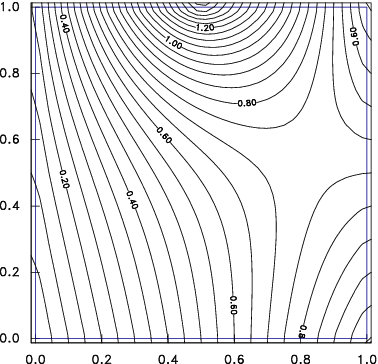Figure 4.4: An elliptic equation, such as Laplace's equation has boundary conditions applied round an entire closed surface. In this case, the potential is prescribed everywhere on the square domain boundary, and contours of potential are plotted.
Alternatively, are the conditions applied on the boundary of a closed domain (as illustrated in Fig. 4.4)? If so (elliptic equations) then the solution everywhere depends upon all the boundary information, it is called a boundary value problem. Then we generally can't successfully integrate from the boundary. "Shooting" doesn't work in multiple dimensions; so we can't turn the problem into an interative initial value problem the way we did in one-dimension. We have to converge to the full solution everywhere simultaneously.

## 4.3  Finite Difference Partial Derivatives

As with ordinary differential equations, a key step in implementing numerical solution of partial differential equations is the expression of the derivatives in terms of the discrete numerical representation of the variables. There are many possible such discrete representations. One might be in terms of the Fourier coefficients ${a}_{\mathit{ij}}$ of a discrete Fourier representation such as:
 $\mathit{\psi }=\sum _{i,j}{a}_{\mathit{ij}}\mathrm{sin}\left(\mathit{\pi }ix/{L}_{x}\right)\mathrm{sin}\left(\mathit{\pi }jy/{L}_{y}\right),$ $\left(4.16\right)$
(where we've chosen to rule out cosine terms by presuming the boundary conditions to be $\mathit{\psi }=0$ at $x=0,{L}_{x}$ or $y=0,{L}_{y}$). There are some equations in which it is computationally efficient to work in terms of such coefficients, especially in coordinate directions that are ignorable. Far more often, though, the representation of our variables is on a discrete spatial mesh with finite spacing in the independent variables.
A mesh is considered to be "structured" if the points at which the solution is to be found are in a regular order in each of the coordinate directions. The most obvious choice is cartesian coordinates. The mesh nodes would then be at positions $\left({x}_{n},{y}_{m},{z}_{l}\right)$, where each array ${x}_{n}$, ${y}_{m}$, etc., is ordered ${x}_{n-1}<{x}_{n}<{x}_{n+1}$, etc. The mesh is then "uniform" if the spacing between nodes is the same for all nodes: ${x}_{n+1}-{x}_{n}=\mathit{\Delta }x$ for all $n$, and similarly for $y$, $z$. Structured meshes are also possible on general curvilinear coordinates, for example cylindrical or spherical coordinates, provided the domain remains "rectangular" in the coordinates; in other words, provided for some set of coordinates $\left(\mathit{\xi },\mathit{\eta },\mathit{\zeta }\right)$, the ${\mathit{\xi }}_{n}$ are independent of ${\mathit{\eta }}_{m}$ and ${\mathit{\zeta }}_{l}$, and so on. Fig. 4.5 gives two examples. For such structured meshes, the most natural and adaptable finite representation is by finite differences.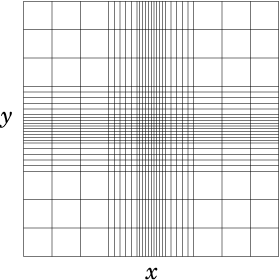(a)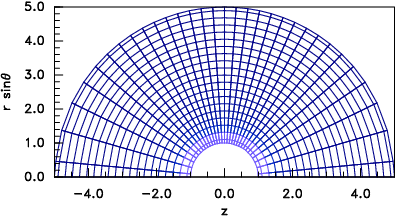(b)
Figure 4.5: Structured grids. (a) Rectangular, but non-uniform. (b) Curvilinear, but still structured.
By contrast, an unstructured grid can have any degree of connectedness. Its cells will not generally be quadrilaterals (in two-dimensions) or hexahedrons (in three dimensions) and it is far less clear how to construct a finite-difference scheme. Fig. 4.6 shows an example of a triangular unstructured mesh${}^{25}$.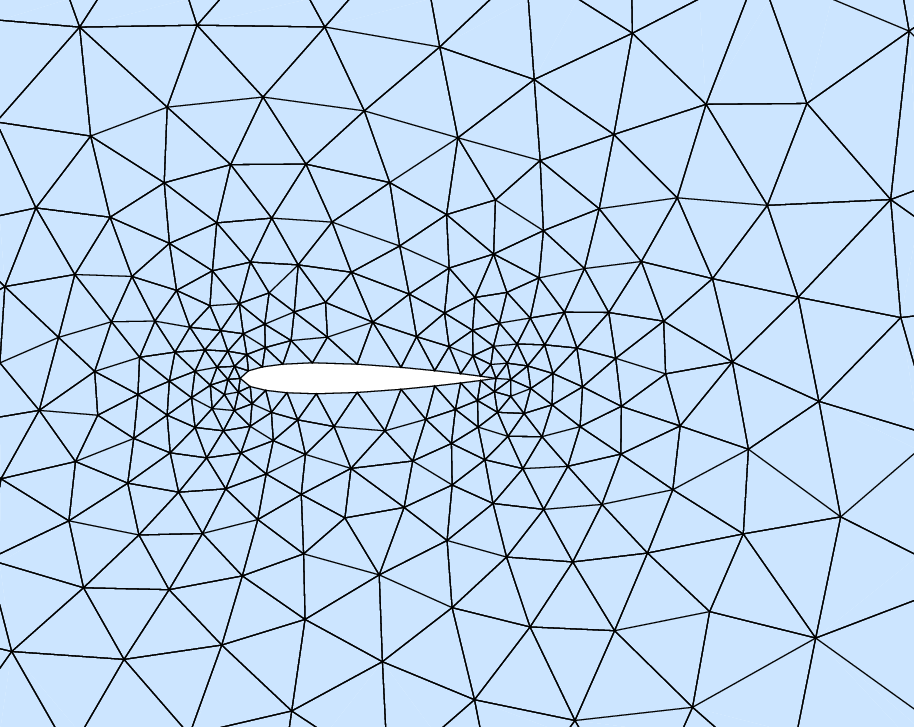Figure 4.6: A region of an unstructured mesh. Such meshes are designed to give greater resolution in areas of more rapid variation, often close to boundaries of complicated or subtle shapes. This example uses a triangular mesh but other choices are possible.
We'll stick to structured meshes here.
Just as in one dimension we use a representation
 ${\frac{d\mathit{\psi }}{\mathit{dx}}|}_{n+1/2}=\frac{{\mathit{\psi }}_{n+1}-{\mathit{\psi }}_{n}}{{x}_{n+1}-{x}_{n}},$ $\left(4.17\right)$
so in two dimensions we can generalize this to partial derivatives of a dependent variable $\mathit{\psi }\left(x,y\right)$ in $x$ and $y$ on mesh positions $n,m$ as
 ${\frac{\partial \mathit{\psi }}{\partial x}|}_{n+1/2,m}=\frac{{\mathit{\psi }}_{n+1,m}-{\mathit{\psi }}_{n,m}}{{x}_{n+1}-{x}_{n}},\mathrm{ }{\frac{\partial \mathit{\psi }}{\partial y}|}_{n,m+1/2}=\frac{{\mathit{\psi }}_{n,m+1}-{\mathit{\psi }}_{n,m}}{{y}_{m+1}-{y}_{m}},$ $\left(4.18\right)$
with obvious generalization to higher dimensions. Two (or more) indices denote the mesh node (or half-node position) where the value of the variable is to be considered evaluated.
Higher derivatives are formed by taking appropriate differences of derivative expressions. So
 ${\frac{{\partial }^{2}\mathit{\psi }}{\partial {x}^{2}}|}_{n,m}=\frac{\left({\frac{\partial \mathit{\psi }}{\partial x}|}_{n+1/2,m}-{\frac{\partial \mathit{\psi }}{\partial x}|}_{n-1/2,m}\right)}{{x}_{n+1/2}-{x}_{n-1/2}}$ $\left(4.19\right)$
and
 ${\frac{{\partial }^{2}\mathit{\psi }}{\partial {y}^{2}}|}_{n,m}=\frac{\left({\frac{\partial \mathit{\psi }}{\partial y}|}_{n,m+1/2}-{\frac{\partial \mathit{\psi }}{\partial y}|}_{n,m-1/2}\right)}{{y}_{m+1/2}-{y}_{m-1/2}}$ $\left(4.20\right)$
into which we substitute the expressions from eq. (4.18). That leads to a sum of coefficients times the values of $\mathit{\psi }$ at three mesh points.
If we are dealing, for example, with Poisson's equation, ${\nabla }^{2}\mathit{\psi }=\mathit{\rho }$, then the entire finite difference expression becomes:
 ${\frac{{\partial }^{2}\mathit{\psi }}{\partial {x}^{2}}|}_{n,m}+{\frac{{\partial }^{2}\mathit{\psi }}{\partial {y}^{2}}|}_{n,m}=\sum _{i=\mathit{adjacent}}{a}_{i}\left({\mathit{\psi }}_{i}-{\mathit{\psi }}_{n,m}\right)={\mathit{\rho }}_{n,m},$ $\left(4.21\right)$
where the sum is over the nodes adjacent to $n,m$, in other words, $i$ takes the four cases $\left(n-1,m\right)$, $\left(n+1,m\right)$, $\left(n,m-1\right)$, $\left(n,m+1\right)$, and for uniform mesh the coefficients ${a}_{i}$ are $1/\mathit{\Delta }{x}^{2}$ or $1/\mathit{\Delta }{y}^{2}$. Written out in full

This form naturally generalizes immediately to higher dimensions. It has a standard structure represented by eq. (4.21), namely that the second order differential operator is represented by the sum over all the adjacent nodes of coefficients times the adjacent values, minus the sum of all the coefficients times the central value. This sum is called a "stencil", representing the differential. The sum of all its coefficients (including the coefficient of the central value) is zero, because if $\mathit{\psi }$ is uniform, ${\nabla }^{2}\mathit{\psi }$ is zero. Written out geometrically for two dimensions the coefficients form a cross pattern which for the uniform-mesh Laplacian is:
 $\begin{array}{cccc}\hfill m+1\hfill & \hfill .\hfill & \hfill 1/\mathit{\Delta }{y}^{2}\hfill & \hfill .\hfill \\ \hfill m\hfill & \hfill 1/\mathit{\Delta }{x}^{2}\hfill & \hfill -\left(2/\mathit{\Delta }{x}^{2}+2/\mathit{\Delta }{y}^{2}\right)\hfill & \hfill 1/\mathit{\Delta }{x}^{2}\hfill \\ \hfill m-1\hfill & \hfill .\hfill & \hfill 1/\mathit{\Delta }{y}^{2}\hfill & \hfill .\hfill \\ \hfill \hfill & \hfill n-1\hfill & \hfill n\hfill & \hfill n+1\hfill \end{array}$ $\left(4.23\right)$
Other linear second order differential operators, or non-uniform or curvilinear meshes, will have different coefficients, but will still have the same geometric shape, and still have the central coefficient equal to minus the sum of the others.
The stencil represented by eq. (4.23), is called a "star stencil". It includes only the adjacent point along coordinate directions. It is second order accurate. One can make differential operator approximations whose errors are of higher order accuracy. Such modified stencils might fill in the corners of the $3×3$ matrix or even go additional steps beyond the edges, using appropriate coefficients. Although there may be specific applications in which such expanded stencils are appropriate, they are rather rare. The main focus is generally on ensuring that the coefficients are calculated well centered so as to maintain the second order accuracy. This is not automatic in non-uniform mesh cases.

## Worked Example: Cylindrical Differences

Determine the type classification of the following partial differential equation, governing a problem in cylindrical coordinates $r,\mathit{\theta }$.
 ${\nabla }^{2}\mathit{\psi }\equiv \frac{1}{r}\frac{\partial }{\partial r}\left(r\frac{\partial \mathit{\psi }}{\partial r}\right)+\frac{1}{{r}^{2}}\frac{{\partial }^{2}\mathit{\psi }}{\partial {\mathit{\theta }}^{2}}=-{k}^{2}\mathit{\psi }.$ $\left(4.24\right)$
Obtain an appropriate difference stencil representing the differential operator, at grid point ${r}_{n}$, ${\mathit{\theta }}_{m}$ for a grid with uniform mesh in $r$ and $\mathit{\theta }$ such that ${r}_{n}=n\mathit{\Delta }r$, and ${\mathit{\theta }}_{m}=m\mathit{\Delta }\mathit{\theta }$.

The quadratic form in $x$ and $y$ arising from the coefficients for the derivatives with respect to $r$ and $\mathit{\theta }$ is
 ${x}^{2}+\frac{1}{{r}^{2}}{y}^{2}+\frac{1}{r}x=\mathit{const}.$ $\left(4.25\right)$
Since ${r}^{2}$ is always positive, this is the equation of an ellipse (the ratio of its axes is $1/r$). The differential equation is elliptic. We can apply a condition around an entire closed boundary in the $r$-$\mathit{\theta }$ plane. In practice $\mathit{\theta }$ is periodic, so there usually isn't a true boundary at $\mathit{\theta }=0$. At $r=0$ (if the domain extends to it), $\frac{\partial \mathit{\psi }}{\partial r}=0$. The boundary conditions are applied at fixed $r$-positions.
To obtain the stencil, start by writing down the first order partial derivatives in the coordinate directions:
 ${\frac{\partial \mathit{\psi }}{\partial r}|}_{n+1/2,m}=\frac{{\mathit{\psi }}_{n+1,m}-{\mathit{\psi }}_{n,m}}{{r}_{n+1}-{r}_{n}},\mathrm{ }{\frac{\partial \mathit{\psi }}{r\partial \mathit{\theta }}|}_{n,m+1/2}=\frac{{\mathit{\psi }}_{n,m+1}-{\mathit{\psi }}_{n,m}}{{r}_{n}\left({\mathit{\theta }}_{m+1}-{\mathit{\theta }}_{m}\right)}.$ $\left(4.26\right)$
Then substitute into the second-derivative forms:
 $\begin{array}{ccc}\multicolumn{1}{c}{\frac{1}{r}\frac{\partial }{\partial r}}& {\left(r\frac{\partial \mathit{\psi }}{\partial r}\right)}_{n,m}\hfill & =\frac{1}{{r}_{n}}\left({r}_{n+1/2}\frac{{\mathit{\psi }}_{n+1,m}-{\mathit{\psi }}_{n,m}}{{r}_{n+1}-{r}_{n}}-{r}_{n-1/2}\frac{{\mathit{\psi }}_{n,m}-{\mathit{\psi }}_{n-1,m}}{{r}_{n}-{r}_{n-1}}\right)\frac{1}{{r}_{n+1/2}-{r}_{n-1/2}}\hfill \\ \multicolumn{1}{c}{}& =\hfill & \left[\left(1+\frac{1}{2n}\right)\left({\mathit{\psi }}_{n+1,m}-{\mathit{\psi }}_{n,m}\right)-\left(1-\frac{1}{2n}\right)\left({\mathit{\psi }}_{n,m}-{\mathit{\psi }}_{n-1,m}\right)\right]\frac{1}{\mathit{\Delta }{r}^{2}}\hfill \\ \multicolumn{1}{c}{}& =\hfill & \left[\left(1+\frac{1}{2n}\right){\mathit{\psi }}_{n+1,m}-2{\mathit{\psi }}_{n,m}+\left(1-\frac{1}{2n}\right){\mathit{\psi }}_{n-1,m}\right]\frac{1}{\mathit{\Delta }{r}^{2}}.\hfill & \hfill \left(4.27\right)\end{array}$

and
 $\begin{array}{ccc}\multicolumn{1}{c}{{\frac{1}{{r}^{2}}\frac{{\partial }^{2}\mathit{\psi }}{\partial {\mathit{\theta }}^{2}}|}_{n,m}}& =\hfill & \frac{1}{{r}_{n}^{2}}\left(\frac{{\mathit{\psi }}_{n,m+1}-{\mathit{\psi }}_{n,m}}{{\mathit{\theta }}_{m+1}-{\mathit{\theta }}_{m}}-\frac{{\mathit{\psi }}_{n,m}-{\mathit{\psi }}_{n,m-1}}{{\mathit{\theta }}_{m}-{\mathit{\theta }}_{m-1}}\right)\frac{1}{{\mathit{\theta }}_{m+1/2}-{\mathit{\theta }}_{m-1/2}}\hfill \\ \multicolumn{1}{c}{}& =\hfill & \left({\mathit{\psi }}_{n,m+1}-2{\mathit{\psi }}_{n,m}+{\mathit{\psi }}_{n,m-1}\right)\frac{1}{{r}_{n}^{2}\mathit{\Delta }{\mathit{\theta }}^{2}}.\hfill & \hfill \left(4.28\right)\end{array}$

Then we see that the required stencil for the differential operator is
 $\begin{array}{cccc}\hfill m+1\hfill & \hfill .\hfill & \hfill 1/\left({r}_{n}\mathit{\Delta }\mathit{\theta }{\right)}^{2}\hfill & \hfill .\hfill \\ \hfill m\hfill & \hfill \left(1-\frac{1}{2n}\right)/\mathit{\Delta }{r}^{2}\hfill & \hfill -2\left[1/\mathit{\Delta }{r}^{2}+1/\left({r}_{n}\mathit{\Delta }\mathit{\theta }{\right)}^{2}\right]\hfill & \hfill \left(1+\frac{1}{2n}\right)/\mathit{\Delta }{r}^{2}\hfill \\ \hfill m-1\hfill & \hfill .\hfill & \hfill 1/\left({r}_{n}\mathit{\Delta }\mathit{\theta }{\right)}^{2}\hfill & \hfill .\hfill \\ \hfill \hfill & \hfill n-1\hfill & \hfill n\hfill & \hfill n+1\hfill \end{array}.$ $\left(4.29\right)$

## Exercise 4. Partial Differential Equations.

1. Determine whether the following partial differential equations, in which $p$ and $q$ are arbitrary real constants, are elliptic, parabolic, or hyperbolic.
(a) ${p}^{2}\frac{{\partial }^{2}\mathit{\psi }}{\partial {x}^{2}}+{q}^{2}\frac{{\partial }^{2}\mathit{\psi }}{\partial {y}^{2}}=0$
(b) ${p}^{2}\frac{{\partial }^{2}\mathit{\psi }}{\partial {x}^{2}}-{q}^{2}\frac{{\partial }^{2}\mathit{\psi }}{\partial {y}^{2}}=\mathit{\psi }$
(c) $\frac{{\partial }^{2}\mathit{\psi }}{\partial {x}^{2}}+4\frac{{\partial }^{2}\mathit{\psi }}{\partial x\partial y}+\frac{{\partial }^{2}\mathit{\psi }}{\partial {y}^{2}}=0$
(d) $\frac{{\partial }^{2}\mathit{\psi }}{\partial {x}^{2}}+2\frac{{\partial }^{2}\mathit{\psi }}{\partial x\partial y}+\frac{{\partial }^{2}\mathit{\psi }}{\partial {y}^{2}}=0$
(e) $\frac{{\partial }^{2}\mathit{\psi }}{\partial {x}^{2}}+p\frac{\partial \mathit{\psi }}{\partial y}=\mathit{\psi }$
(f) $\frac{{\partial }^{2}\mathit{\psi }}{\partial {x}^{2}}+\frac{{\partial }^{2}\mathit{\psi }}{\partial {y}^{2}}+\frac{{\partial }^{2}\mathit{\psi }}{\partial {z}^{2}}=0$
(g) $p\frac{\partial \mathit{\psi }}{\partial x}+\mathit{qy}\frac{\partial \mathit{\psi }}{\partial y}=1$

2. Write a computer code function${}^{26}$ to evaluate the difference stencil in two dimensions for the anisotropic partial differential operator, $L=\frac{{\partial }^{2}}{\partial {x}^{2}}+2\frac{{\partial }^{2}}{\partial {y}^{2}}$. The code function is to operate on a quantity $f\left(x,y\right)={f}_{\mathit{ij}}$, represented as a matrix of the values at discrete points on a structured, equally-spaced, 2-D mesh with ${N}_{x}$ and ${N}_{y}$ nodes in the $x$ and $y$ directions, spanning the intervals $0\le x\le {L}_{x}$, $0\le y\le {L}_{y}$. The function should accept parameters ${N}_{x},{N}_{y},{L}_{x},{L}_{y},i,j,f$ and return the corresponding finite-difference expression for ${g}_{\mathit{ij}}=Lf$ at mesh point $i,j$.
Write also a test program to construct $f\left(x,y\right)=\left({x}^{2}+{y}^{2}/2\right)$ on the mesh nodes, giving ${f}_{\mathit{ij}}$, and call your stencil function, with $f$ and the corresponding ${N}_{x},{N}_{y},{L}_{x},{L}_{y}$ as arguments, to evaluate ${g}_{\mathit{ij}}$ and print it.
Submit the following as your solution:
1. Your code in a computer format that is capable of being executed, citing the language it is written in.
2. A brief answer to the following. Will your function work at the boundaries, $x=0,{L}_{x}$, or $y=0,{L}_{y}$? If not, what is needed to make it work there?
3. The values of ${g}_{\mathit{ij}}$ for four different nodes corresponding to two different interior $i$ and two different interior $j$, when ${N}_{x}={N}_{y}=10$, ${L}_{x}={L}_{y}=10$.
4. Brief answer to: Are there inefficiencies in using a code like this to evaluate $Lf$ everywhere on the mesh? If so, how might those inefficiencies be avoided?